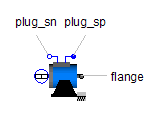Hydraulic Cylinder - MapleSim Help

Hydraulic Cylinder

Ideal transformation between hydraulic and 1-D translational workDescription The Hydraulic Cylinder component models an ideal translational actuator. It transforms energy between the hydraulic and translational domains.Equations $p={p}_{a}$ $q={q}_{a}=A\frac{\mathrm{d}{s}_{\mathrm{rel}}}{\mathrm{d}t}$ $f=-{f}_{a}={f}_{b}=-Ap$ ${s}_{\mathrm{rel}}={s}_{b}-{s}_{a}$Variables

 Name Units Description Modelica ID $p$ $\mathrm{Pa}$ Pressure in cylinder p $q$ $\frac{{m}^{3}}{s}$ Flow rate into cylinder q ${s}_{\mathrm{rel}}$ $m$ Relative distance between flanges s_rel $f$ $N$ Force between flanges f ${f}_{x}$ $N$ Force at flange $x,x\in \left\{a,b\right\}$between flanges flange_x.fConnections

 Name Description Modelica ID $\mathrm{portA}$ Hydraulic port portA ${\mathrm{flange}}_{a}$ Left flange of compliant 1-dim. translational component flange_a ${\mathrm{flange}}_{b}$ Right flange of compliant 1-dim. translational component flange_bParameters

 Name Default Units Description Modelica ID $A$ $1$ ${m}^{2}$ Cross-sectional area of piston A